• matlab开发-状态空间传递函数。该代码演示了如何将MIMO系统从状态空间形式转换为传递函数形式。Simulink基础
• 实验一 MATLAB 系统的传递函数状态空间表达式的 换 一 实验目的 1学习多变量系统状态空间表达式的建立方法 2 通过编程上机调试掌握多变量系统状态空间表达式与传递函数之间相互 转换的方法 3掌握相应的MATLAB ...
• Transformation of Mathematical Models with MATLAB 第一：由传递函数变换为状态空间表达式 假设系统的传递函数如下： 利用MATLAB自带的函数：tf2ss得到状态空间表达式，代码如下： 得到的...
数学模型：闭环传递函数与状态空间之间的相互转换

Transformation of Mathematical Models with MATLAB

第一：由传递函数变换为状态空间表达式

假设系统的传递函数如下：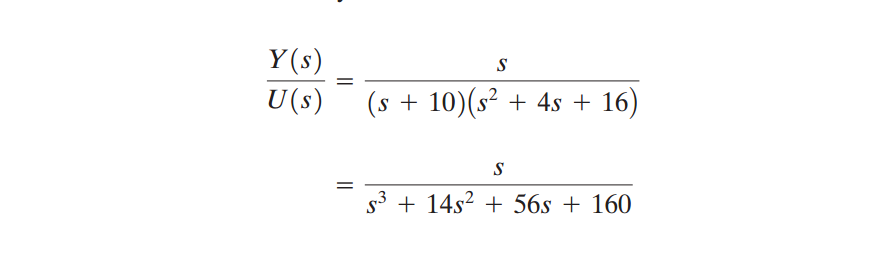利用MATLAB自带的函数：tf2ss得到状态空间表达式，代码如下：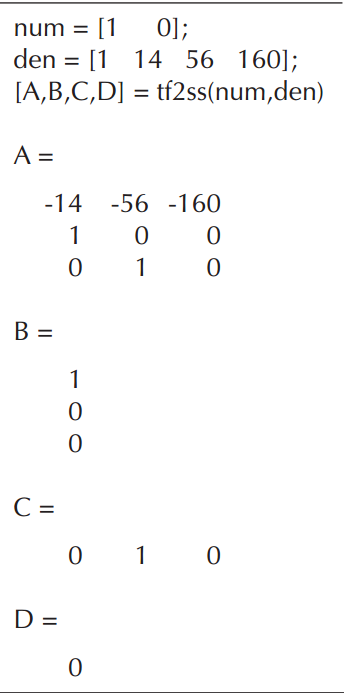得到的状态空间表达式形式如下：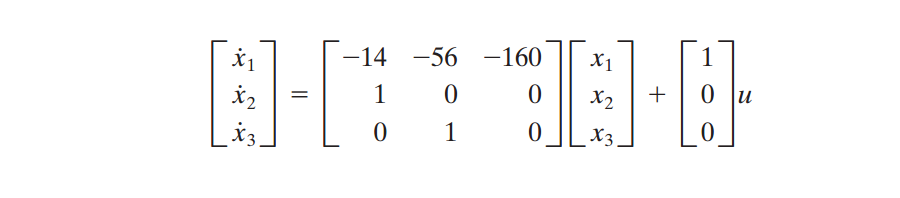第二：由状态空间表达式变换为传递函数

假设系统的状态空间表达式如下：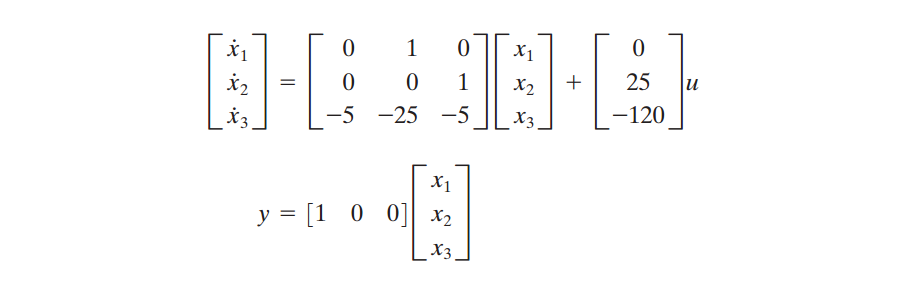利用MATLAB自带的函数：ss2tf得到状态空间表达式，代码如下：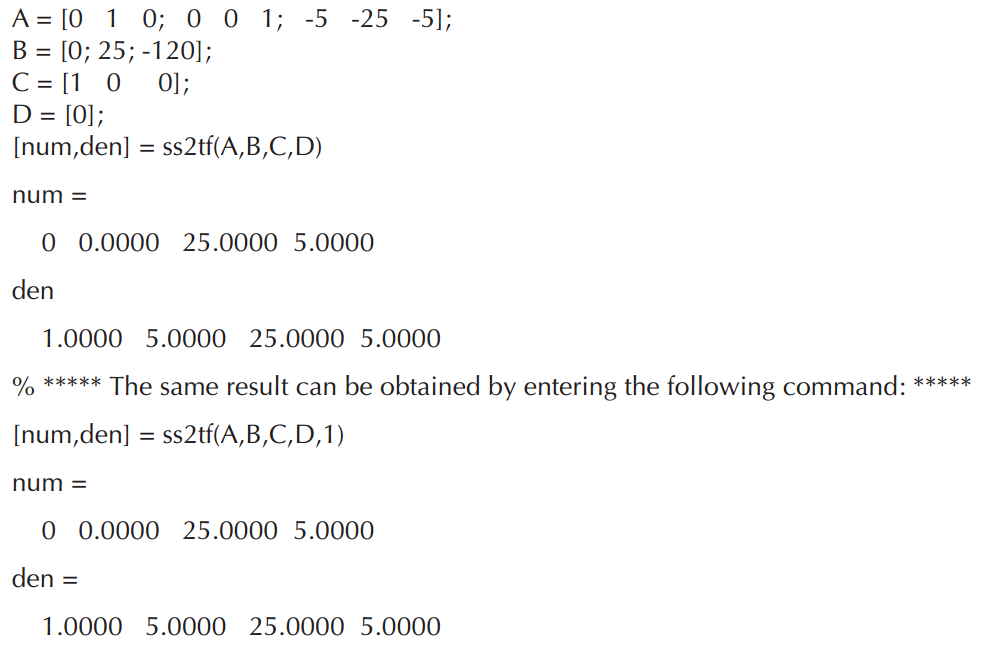得到的传递函数形式如下：展开全文• 传递函数：在零初始条件下，线性定常系统输出象函数X0(s)与输入象函数Xi(s)之比，称为系统的传递函数，用G(s)表示。 传递函数的概念只适用于线性定常系统。 状态空间模型：系统的动态特性由状态变量构成的一阶...
传递函数：在零初始条件下，线性定常系统输出象函数X0(s)与输入象函数Xi(s)之比，称为系统的传递函数，用G(s)表示。

传递函数的概念只适用于线性定常系统。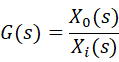状态空间模型：系统的动态特性由状态变量构成的一阶微分方程组（状态空间表达式）来描述，能同时给出系统全部独立变量的响应，因而能同时确定系统发全部内部运动状态。状态空间模型即状态空间表达式，包括状态方程和输出方程，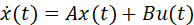，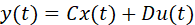，A：系统矩阵，B：输入矩阵，C：输出矩阵，D：直传矩阵

状态空间表达式构成对一个系统动态行为的完整描述。

MATLAB对于控制方面的问题可以很好的进行仿真

1、传递函数变换为状态空间方程，其调用格式为：[A,B,C,D]=tf2ss(num,den)

例：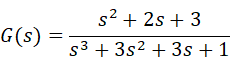，num=[0,1,2,3]，den=[1,3,3,1]，[A,B,C,D]=tf2ss(num,den)

2、状态空间方程变换为传递函数，其调用格式为：[num,den]=ss2tf(A,B,C,D,iu)，iu表示多输入时输入的编号

3、利用matlab由状态空间表达式建立状态空间模型，其调用格式为：

sys=ss(A,B,C,D)，sys为连续系统的状态空间模型；

sys=ss(A,B,C,D,Ts)，sys为离散系统的状态空间模型，Ts为采样时间。

4、利用matlab由传递函数建立状态空间模型，其调用格式为：sys=tf(num,den)

5、任意输入和初始状态下系统响应的调用格式：

[y,t,x]=lsim(sys,u,t,x0)，u为输入，t为时间（0:0.01:3），x0为初始状态

6、对系统离散化的调用格式：

[G,H]=czd(A,B,Ts)，Ts为采样周期


展开全文• 状态空间模型转换成传递函数模型 例：matlab程序如下 A=[-21,19,-20;19,-21,20;40,-40,-40]; B=[0,1,2]’; C=[1,0,2]; D=; sf=ss(A,B,C,D); g=tf(sf) 结果： g = 4 s^2 + 67 s + 1640 s^3 + 82 s^2 + 3360 s +...
现代控制理论 用matlab将状态空间模型转换成传递函数模型（便于求各种响应）
例：matlab程序如下
A=[-21,19,-20;19,-21,20;40,-40,-40];
B=[0,1,2]’;
C=[1,0,2];
D=;
sf=ss(A,B,C,D);
g=tf(sf)
结果：
g =
  4 s^2 + 67 s + 1640


s^3 + 82 s^2 + 3360 s + 6400
Continuous-time transfer function.


展开全文•    传递函数状态空间之间可相互转换，可以使用的matlab函数有 [A,B,C,D] = tf2ss(NUM,DEN) [NUM,DEN] = ss2tf(A,B,C,D,iu)    传递函数的形式唯一，但状态空间的形式不唯一，可以有多种。 1、一阶惯性...
   传递函数与状态空间之间可相互转换，可以使用的matlab函数有
[A,B,C,D] = tf2ss(NUM,DEN)
[NUM,DEN] = ss2tf(A,B,C,D,iu)

传递函数的形式唯一，但状态空间的形式不唯一，可以有多种。
1、一阶惯性环节时间常数为T，本身为低通滤波器，截止频率wc为1/T，通过伯德图可查看，如设置T=0.1，则
T = 0.1;
sys = tf(1,[T 1]);
bode(sys);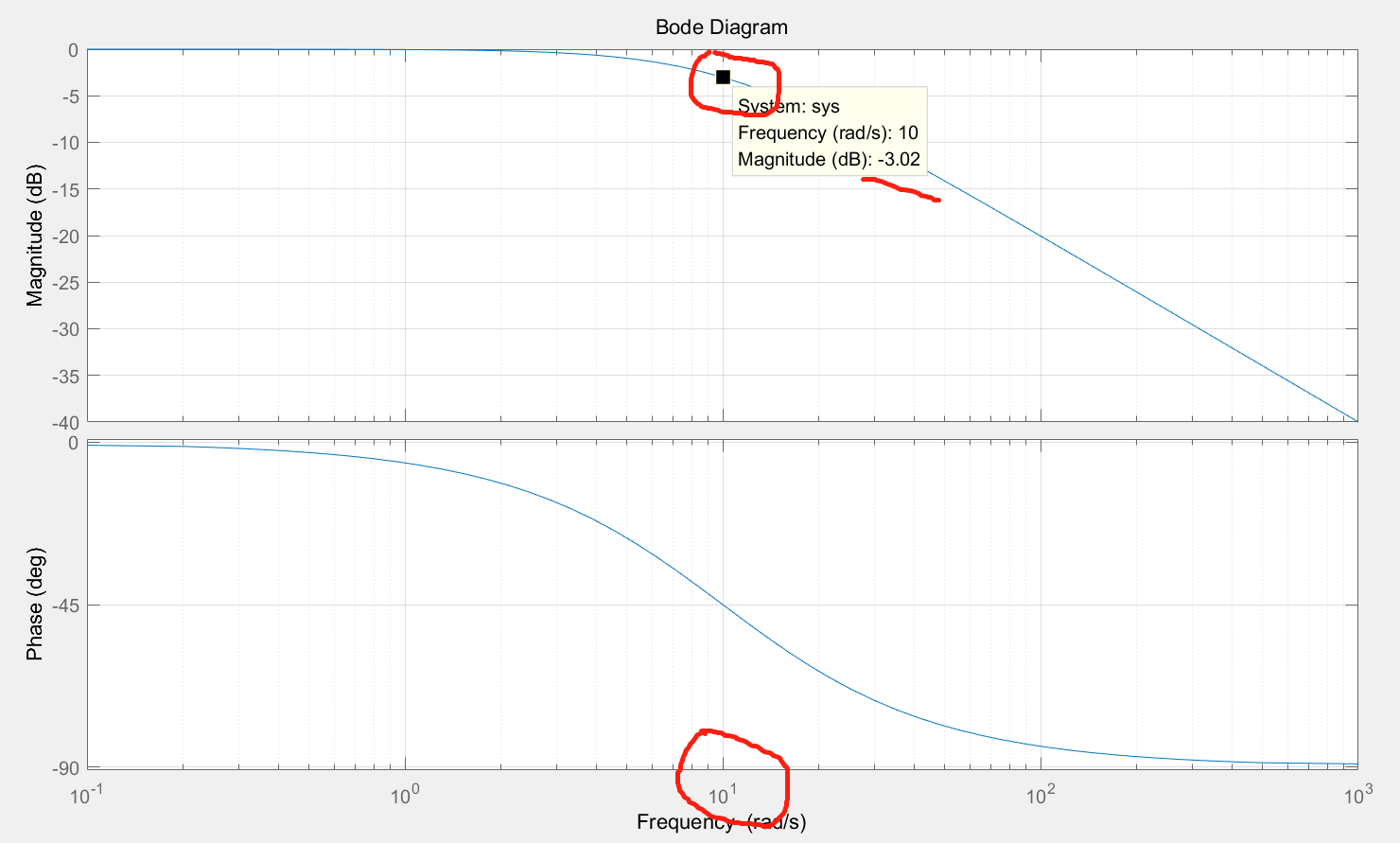a. 离散形式
设u为输入，x为输出，h为步长，则：simulink为：b.状态空间形式形式1：
		A = -1/T;
B = 1/T;
C = 1;
D = 0;

即：形式2：
		A = -1/T;
B = 1;
C = 1/T;
D = 0;

即：c.仿真仿真结果：传递函数、离散形式及状态空间形式所仿真的结果是一致的。
2、二阶震荡环节低通滤波器，当阻尼等于0.707时，传递函数的截止频率wc是wn，如wn等于10，bode图如下：a. 离散形式
设u为输入，x为输出，h为步长，则：simulink为：b. 状态空间
形式1：
		A = [0 1;-wn^2 -2*ksi*wn];
B = [0;wn^2];
C = [1 0];
D = 0;

即：形式2：
		A = [0 1;-wn^2 -2*ksi*wn];
B = [0;1];
C = [wn^2 0];
D = 0;

即：c.仿真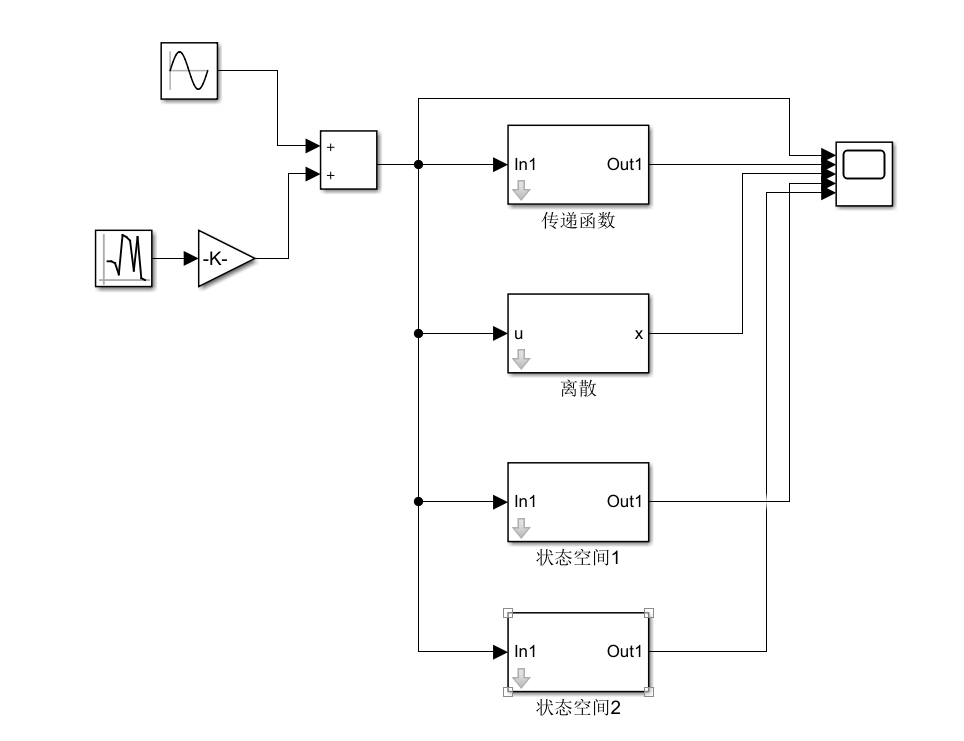仿真结果：传递函数、离散形式及状态空间形式所仿真的结果是一致的。

上面两个仿真的simulink模型在此：
https://download.csdn.net/download/niu_88/11799584

3、传递函数离散化以及状态空间转换
可参考此资料：
https://download.csdn.net/download/niu_88/11799588


展开全文simulink
• 装 订 线 实 验 报 告 实验名称 用 MATLAB分析状态状态空间模型 系 ...法 2 掌握传递函数状态空间表达式之间相互转换的方法学会用 MATLAB实现不同 模型之间的相互转换 3 熟悉系统的连接学会用 MATLAB确定整个系统的状
• 实验一 用MATLAB分析状态空间模型 1实验设备 MATLAB软件 2实验目的 学习系统状态空间表达式的建立方法了解系统状态空间表达式与传递函数相互转换的方法 通过编程上机调试掌握系统状态空间表达式与传递函数相互转换...
• matlabss函数tf函数ss指定状态空间模型或者将线性时不变系统状态空间语法sssys=ss(a,b,c,d)sys=ss(a,b,c,d,Ts)sys=ss(d)sys=ss(a,b,c,d,ltisys)sys_ss=ss(sys)说明ss用来创建实数或复数的状态空间模型，或者将...
• ss指定状态空间模型或者将线性时不变系统状态空间语法sssys = ss(a,b,c,d)sys = ss(a,b,c,d,Ts)sys = ss(d)sys = ss(a,b,c,d,ltisys)sys_ss = ss(sys)说明ss用来创建实数或复数的状态空间模型，或者将传递函数和...
• 2.传递函数状态空间之间的相互转换 [A,B,C,D]=tf2ss(,[1,25,0]) [num,den]=ss2tf(A,B,C,D,1) 3.如何获取状态空间的A\B\C\D [A,B,C,D]=ssdata(sys) 4.连续状态空间的离散化 sys1=c2d(sys,ts,'z') ...
• ss指定状态空间模型或者将线性时不变系统状态空间语法sssys = ss(a,b,c,d)sys = ss(a,b,c,d,Ts)sys = ss(d)sys = ss(a,b,c,d,ltisys)sys_ss = ss(sys)说明ss用来创建实数或复数的状态空间模型，或者将传递函数和...
• 控制原理中的Matlab仿真与函数合集1 基本模型1.1 传递函数模型(tf)1.1.1 tf(num,den)1.2 状态空间模型(ss)1.2.1 ss(A,B,C,D)1.2.2 ssdata(sys)1.2.3 canon(sys)1.3 零极点模型(zpk)1.3.1 pzmap(sys)1.4 模型间的转换...
• 要求 考虑传递函数H（z）=KH1（z） H2（z），其中， 　按给定的H1（Z）和H2（z）计算级联滤波器各级的直接II型状态空间表达形式。并计算H（z）的零极点分布。 　欢迎转载，信息来自维库电子市场网...
• 学习系统状态空间表达式的建立方法了解系统状态空间表达式与传递函数相互转换的方法 2.通过编程上机调试掌握系统状态空间表达式与传递函数相互转换方法 3.学习MATLAB的使用方法 实验步骤 1根据所给系统的结构图写出...
• * * * * * * * * 机械工业出版社 CHINA MACHINE PRESS 4.3 基于MATLAB的数学模型转换 4.3.2 传递函数状态空间表达式的相互转换 2. 状态空间表达式与零极点形式的相互转换 例4-14对于例4-13中给出的状态空间表达式...
• 数学模型种类与转换 MATLAB中用四种数学模型表示控制系统传递函 数模型零极点增益模型状态空间模型动态 结构图SIMULINK中使用 实际应用中根据要解决问题的需要往往要进 行不同种类模型之间的转换MATLAB控制系统工 具...
• 函数sos2ss将二阶滤波器节映射为直接II型状态空间形式，而函数sos2tf将一组 2阶滤波器节转换为传递函数。对于滤波器节为1阶的情况，系数b2i和a2i将被没为零。函数sos2zp将2阶滤波器节转换为零点－极点－增益的形式...
• 控制系统常用的数学模型有三种：传递函数、零极点增益和状态空间。每种模型均有连续/离散之分，它们各有特点，有时需在各种模型之间进行转换。本节主要介绍它们的MATLAB表示及三种模型之间的相互转换。 2.8.1连续...parallel 工具 c
• 1、多项式转换为零极点 ...3、状态空间转换成多项式传递函数 [num,den]=ss2tf(a,b,c,d); 4、多项式传递函数转换成状态空间 [a,b,c,d]=tf2ss(num，den); 5、零极点转换成状态...
• 利用数值积分法求siso系统的输出，首先将系统的传递函数转换成状态空间型，然后利用欧拉法求解
• 5．5．2 基于MPC状态空间模型的控制器设计与仿真 5．6 系统分析函数 5．7 模型预测控制工具箱功能函数 第6章 模糊逻辑工具箱 6．1 模糊逻辑理论简介 6．1．1 模糊集合 6．1．2 模糊关系 6．1．3 模糊推理 6．2 ...
• 1. 创建系统的传递函数模型 ;举例使用tf命令;引用Laplace变量s的多项;2.创建零极点-增益模型 zp;举例;3.创建连续系统状态空间模型 ;PowerPoint 演示文稿;举例;4. 创建FRD模型 frd;举例;各模型之间的相互转换;5获
• 实验二MATLAB系统模型... 实验仪器 实验仪器 计算机 三实验内容 控制系统地模型 控制系统地表示可用三种模型传递函数零极点增益状态空间 ?每一种模型又有连续 与离散之分?为分析系统方便有时需要在三种模型间转换 .M
• MATLAB控制系统工具箱中提供了函数命令ss2tf(), 可以把给定的状态空间模型转换为传递函数模型。 调用格式：[num,den] = ss2tf(A,B,C,D,1) 其中最后一个1表示单输入。 示例： 运行代码 A = [0 1 0; 0 0 1; -5 -25 -5]...
• 1.6MATLAB/Simulink在状态空间中的应用 1.7MATLAB在PID控制器设计中的应用 1.8MATLAB在导弹系统中的应用 第2章通信系统建模与仿真 2.1数字信号的传输 2.1.1数字信号的基带传输 2.1.2数字信号的载波传输 2.2...
• 12.2.2离散传递函数(Z传递函数) 12.2.3离散状态空间模型 12.3数学模型的MATLAB相关函数 12.3.1传递模型的函数 12.3.2零极点模型函数 12.3.3状态空间模型函数 第13章径向基网络算法分析与实现 13.1径向基网络...
• 本期视频时长约70分钟，通过具体的系统分析案例，详细地讲解了连续系统的三种时域分析方法：系统微分方程求解法，基于传递函数的lsim求解方法，基于冲激响应的卷积积分求解法。视频的最后，还对各种方法进行了总结和...
• 电力电子系统依靠反馈控制将来自电源的电压...由于该理论基于线性时不变(LTI)系统，例如传递函数状态空间模型，因此要将其应用于电力电子系统，工程师需要找到此类系统的LTI表示形式。频率响应估计(也称为AC扫描)...# matlab状态空间转传递函数matlab 订阅